Line of Best Fit

 A line of best fit  (or "trend" line) is a straight line that best represents the data on a scatter plot.  This line may pass through some of the points, none of the points, or all of the points.

You can examine "lines of best fit" with:
1.  paper and pencil only
2.  a combination of graphing calculator and
paper and pencil
3.  or solely with the graphing calculator

 Sandwich Total Fat (g) Total Calories Hamburger 9 260 Cheeseburger 13 320 Quarter Pounder 21 420 Quarter Pounder with Cheese 30 530 Big Mac 31 560 Arch Sandwich Special 31 550 Arch Special with Bacon 34 590 Crispy Chicken 25 500 Fish Fillet 28 560 Grilled Chicken 20 440 Grilled Chicken Light 5 300

Example:
Is there a relationship between the fat grams and the total calories in fast food?
(refer to the data at the left)Paper and Pencil Solution:
 See the bottom of this page for how to also do "my choice" line of best fit on the calculator.
 1.  Prepare a scatter plot of the data on graph paper. 2.  Using a strand of spaghetti, position the spaghetti so that the plotted points are as close to the strand as possible. Bibs, the cat, is assisting with the spaghetti alignment for this example.3.  Find two points that you think will be on the "best-fit" line.  Perhaps you chose the points
(9, 260) and (30, 530).  Different people may choose different points.

4.  Calculate the slope of the line through your two points (rounded to three decimal places).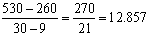5.  Write the equation of the line.  This equation can now be used to predict information that was not plotted in the scatter plot.  For example, you can use the equation to find the total calories based upon 22 grams of fat.

 Equation: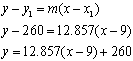or  y = 12.857x + 144.287 Prediction based on 22 grams of fat:Different people may choose different points and arrive at different equations.  All of these equations are "correct", but which one is actually the "best"?  To determine the actual "best" fit, we will use a graphing calculator.

Graphing Calculator Solution:
 1.  Enter the data into Lists and Spreadsheets. Column A will be labeled "fat" (for the fat grams) and column B will be labeled "cal" (for the calories). See Lists and Spreadsheets for how to enter data.2.  Graph the scatter plot. From, choose #5 Data and Statistics. Hit ENTER.
Using the Nav Pad, arrow to the bottom of the screen and choose the x-variable to be "fat". Then move to the left side of the screen and choose the y-variable to be "cal". You will now see your scatter plot.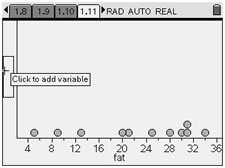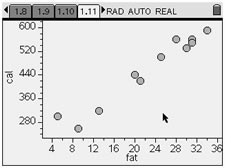3. NOW, have the calculator determine the BEST "line of best fit".
A "line of best fit" is referred to as a linear regression.
From, choose #3 Actions, #5 Regression, and #1Show Linear (mx + b). The graph will appear with the "line of best fit" equation (the linear regression equation).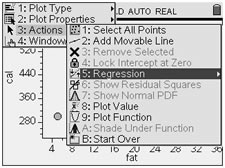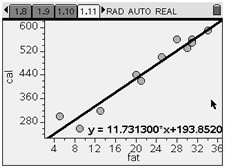Note: You can "grab" any of the points and move them. The equation will change accordingly, as will the spreadsheet entries. Important Note: If your calculator is set to FLOAT, this linear regression equation will be rounded to the nearest integer. If you calculator is set to FLOAT 6 (the default), this linear regression equation will contain 6 decimal places which may contain inaccurate trailing zeros.
Graphing calculator "line of best fit": y = 11.7313x + 193.852
(using default settings on calculator - minus the trailing zeros)

 Paper and Pencil Solution on the Calculator: If you prepare your scatter plot on the Graphs & Geometry page, you can examine "your own" line of best fit on the calculator including its equation. After you enter your data .... From HOME, choose #2 Graphs&Geometry. From MENU, choose #3 Graph Type and #4 Scatter Plot, as seen at the right. Hit ENTER.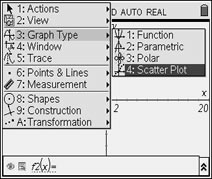At the bottom of the screen, highlight the box naming the set to be used for the x-values, click, and choose "fat". Use your TAB key to highlight box if needed. Do the same for the y set and choose "cal".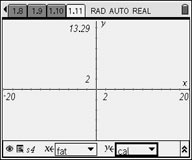From MENU, choose #4 Window and #9 Zoom Data, to set the window for the plot. Hit ENTER.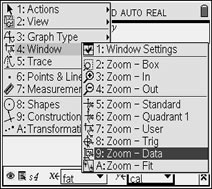In the graph at the right, the Entry Line was hidden to allow for a full view of the graph. To hide entry line: CTRL- G or MENU, View, #6 Entry Line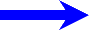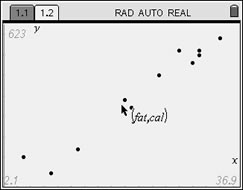As we did with pencil and paper, choose two points that you think will be on the "best-fit" line.  Perhaps you choose again the points (9, 260) and (30, 530).  Different people may still choose different points.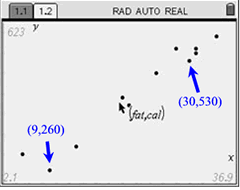From this screen, choose MENU, #6 Points & Lines, #4 LineMove the pencil to the first point of your choice (9,260). Enter.(Should you make a mistake, use CTRL - ESC to undo the mistake.)Move the pencil to the first point of your choice (9,260). Enter. Now, get the equation of the line:Highlight the line. Hit MENU. #1 Action, #6 Coordinates and EquationsRead the equation.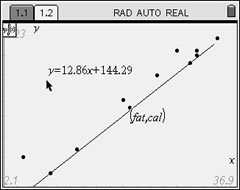Drag the equation so it can be clearly seen.